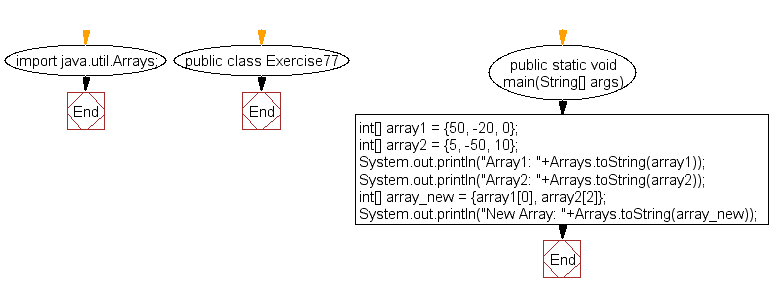﻿ Java: Array with the first and last elements from 2 arrays# Java Exercises: Create a new array of length 2 from two arrays of integers with three elements and the new array will contain the first and last elements from the two arrays

## Java Basic: Exercise-77 with Solution

Write a Java program to create a new array of length 2 from two arrays of integers with three elements and the new array will contain the first and last elements from the two arrays.

Sample Solution:

Java Code:

``````import java.util.Arrays;
public class Exercise77 {
public static void main(String[] args)
{
int[] array1 = {50, -20, 0};
int[] array2 = {5, -50, 10};
System.out.println("Array1: "+Arrays.toString(array1));
System.out.println("Array2: "+Arrays.toString(array2));
int[] array_new = {array1, array2};
System.out.println("New Array: "+Arrays.toString(array_new));
}
}
```
```

Sample Output:

```Array1: [50, -20, 0]
Array2: [5, -50, 10]
New Array: [50, 10]
```

Pictorial Presentation:Flowchart:Java Code Editor:

What is the difficulty level of this exercise?

Test your Programming skills with w3resource's quiz.

﻿

## Java: Tips of the Day

getEnumMap

Converts to enum to Map where key is the name and value is Enum itself.

```public static <E extends Enum<E>> Map<String, E> getEnumMap(final Class<E> enumClass) {
return Arrays.stream(enumClass.getEnumConstants())
.collect(Collectors.toMap(Enum::name, Function.identity()));
}
```

Ref: https://bit.ly/3xXcFZt